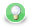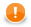### Arithmetic Operators

The arithmetic operators perform basic mathematical operation (addition, subtraction, etc.), concatenate strings or lists or merge content of two maps.

The operators can be used more times in one expression. The result depends on the order of operators within the expressions. In such a case, you can express priority of operations by parentheses.

TipIf you are unsure about priority of operators or associativity, the safest way is to use parentheses.
 Addition + Subtraction and Unitary minus - Multiplication * Division / Modulus % Incrementing ++ Decrementing --

`+`

`numeric type +(`numeric type left, numeric type right`)`;

`string +(`string left, string right`)`;

`list +(`list left, list right`)`;

`map +(`map left, map right`)`;

The operator `+` serves to sum the values of two expressions, concatenate two string values, concatenate two lists or merge content of two maps.

Nevertheless, if you want to add any data type to a string, the second data type is converted to a string automatically and it is concatenated with the first (string) summand. But remember that the string must be on the first place.

Naturally, two strings can be summed in the same way.

Note also that the `concat()` function is faster than `+`. You should use this function instead of adding any summand to a string. See concat.

The addition of two boolean values or two date data types is not possible. To create a new value from two boolean values, you must use logical operators instead.

```integer in01 = 1;
integer in02 = 2;
integer in03 = in02 + in01; // 3

// string concatenation
string s1 = "Hello";
string s2 = " World!";
string s3 = s1 + s2; // Hello World!

decimal price = 1.50d;
string order = "turnip " + price; // turnip 1.50

variant mapVar = {1 -> {2 -> 3}, 4 -> {5 -> 6}};
printLog(info, "mapVar = " + mapVar); // prints "mapVar = {1={2=3}, 4={5=6}}"

// concatenation of two lists
integer [] il1 = ;
integer [] il2 = [3,5];
integer [] il3 = il1 + il2; // [2,3,5]

// merge of two maps
map[string,string] m1;
map[string,string] m2;
map[string,string] m3;
m1["d"] = "Delta";
m1["f"] = "Foxtrot";
m2["e"] = "Echo";
m3 = m1 + m2;```
TipIf you concatenate several strings, use the following approach instead of the plus sign: ```string[] buffer; buffer.append("\n"); buffer.append("\n"); buffer.append("String concatenation example\n"); buffer.append("\n"); // append multiple strings at once buffer.copy(["", "\n", "Sample content", "\n", "", "\n"]); buffer.append(""); // concatenates the list into a single string, null list elements are converted to the string "null" string result = join("", buffer);``` This example is analogous to using a java.lang.StringBuilder. Avoid Schlemiel the Painter's algorithm for concatenation of a large number of strings. You can also use concat or concatWithSeparator to concatenate strings. The difference is that join allows storing intermediate results in a list of strings, while concat requires that all operands are passed as parameters simultaneously.

#### Subtraction and Unitary minus

`-`

`numeric type -(`numeric type left, numeric type right`)`;

The operator `-` subtracts one numeric data type from another.

If the numeric types of operands differ, firstly, automatic conversions are applied and then subtraction is performed.

`integer i1 = 5 - 3;`

#### Multiplication

`*`

`numeric type *(`numeric type left, numeric type right`)`;

The operator `*` multiplies two numbers.

Numbers can be of different data types. If data types of operands differ, automatic conversion is applied.

```integer i1 = 2 * 3;
decimal d1 = 1.5 * 3.5;
double  d2 = 2.5 * 2;```

#### Division

`/`

`numeric type /(`numeric type left, numeric type right`)`;

Operator `/` serves to divide two numeric data types. Remember that you must not divide by zero. Division by zero throws TransformLangExecutorRuntimeException or returns `Infinity` (in the case of `double` (`number`) data type).

```integer i1 = 7  / 2;      // i1 == 3
long    l2 = 9L / 4L;     // l2 == 2L
decimal d3 = 6.75D / 1.5D // d3 == 4.5D
double d4  = 6.25  / 2.5  // d4 == 2.5```

#### Modulus

`%`

`numeric type %(`numeric type left, numeric type right`)`;

Operator `%` returns the remainder of division. The operator can be used for floating-point, fixed-point and integral data types.

```integer in1 = 7 % 3;         // in1 == 1
long    lo1 = 8 % 5;         // lo1 == 3
decimal de1 = 15.75D % 3.5D  // de1 == 1.75D
double  do1 = 6.25 % 2.5     // do1 == 1.25```

#### Incrementing

`++`

Operator `++` serves to increment numeric data type value by one. The operator can be used for both floating-point data types and integer data types.

If it is used as a prefix, the number is incremented first and then it is used in the expression.

If it is used as a postfix, first, the number is used in the expression and then it is incremented.

ImportantRemember that the incrementing operator cannot be applied on literals, record fields, map, or list values of integer data type.
```integer i1 = 20;
integer i2 = ++i1; // i1 = i1 + 1; i2 = i1;     i1 == 21 and i2 == 21
integer i3 = i++   // i3 = i1;     i1 = i1 + 1; i1 == 22 and i3 == 21```

#### Decrementing

`--`

Operator `--` serves to decrement numeric data type value by one.

The operator can be used for floating-point, fixed-point and integral data types.

If it is used as a prefix, the number is decremented first and then it is used in the expression.

If it is used as a postfix, first, the number is used in the expression and then it is decremented.

ImportantRemember that the decrementing operator cannot be applied on literals, record fields, map, or list values of integer data type.
```integer i1 = 20;
integer i2 = --i1; // i1 = i1 - 1; i2 = i1;     i1 == 19 and i2 == 19
integer i3 = i1--; // i3 = i1;     i1 = i1 - 1; i1 == 18 and i3 == 19```